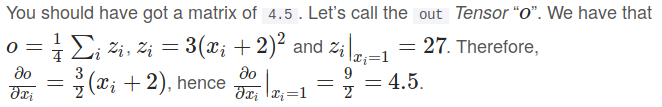The equality 1/4 sum(zi, zi) = 3(xi + 2)^2 is incorrect; it should be 1/4 sum(zi, zi) = 1/4 * 3(xi + 2)^2

Hi,

Which tutorial are you refering to please?

It’s this one.
I think, @chrobles misunderstood.

There is no equality here. i.e., these are two assignment statements separated by a comma.

``````o = 1/4 sum(z_i)
z_i = 3(x_i + 2)^2
``````
1 Like

Thanks for clarifying @InnovArul, that’s correct! My mistake.